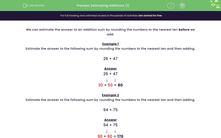### Comprehensive & curriculum aligned

In this worksheet, students will estimate the answer to addition sums by rounding two numbers to the nearest ten and then adding.Key stage:  KS 2

Curriculum topic:   Number: Addition and Subtraction

Curriculum subtopic:   Estimate and Check Answers

Popular topics:   Estimation worksheets

Difficulty level:#### Worksheet Overview

We can estimate the answer to an addition sum by rounding the numbers to the nearest ten before we add.

Remember that a number that ends in five or above rounds up to the next ten.

A number that ends in four or below rounds down to the previous ten.

Example 1

Estimate the answer to the following sum by rounding the numbers to the nearest ten and then adding.

26 + 47

26 + 47

↓      ↓

30 + 50 = 80

Example 2

Estimate the answer to the following sum by rounding the numbers to the nearest ten and then adding.

94 + 75

94 + 75

↓      ↓

90 + 80 = 170

Remember that 75 rounds up to 80, 35 rounds up to 40, 25 rounds up to 30 etc.

Let's have a go at some questions now.### What is EdPlace?

We're your National Curriculum aligned online education content provider helping each child succeed in English, maths and science from year 1 to GCSE. With an EdPlace account you’ll be able to track and measure progress, helping each child achieve their best. We build confidence and attainment by personalising each child’s learning at a level that suits them.

Get started